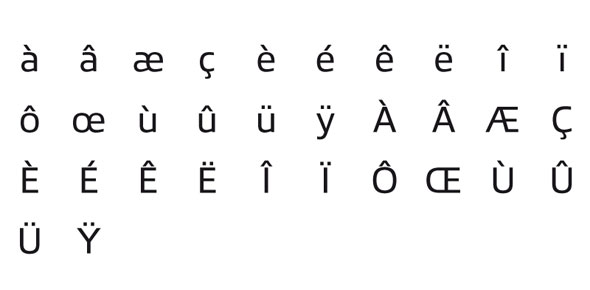# French Ring Rules: Brevet

18 Questions | Total Attempts: 86SettingsTest your knowledge of the rules of French Ring Sport.

• 1.
How many Exercises in Brevet?
• A.

4

• B.

5

• C.

6

• D.

7

• E.

8

• 2.
How many points in Brevet must a dog score in each protection exercises?
• A.

15

• B.

24

• C.

20

• D.

18

• E.

25

• 3.
What is the Minimum score you can score in Brevet and pass?
• A.

75 points

• B.

80 points

• C.

85 points

• D.

90 points

• E.

95 points

• 4.
How many meters is the face attack in Brevet?
• A.

20 meters

• B.

25 meters

• C.

30 meters

• D.

35 meters

• E.

50 meters

• 5.
How long is the dog biting the decoy during the Face attack?
• A.

5 seconds

• B.

10 seconds

• C.

15 seconds

• D.

20 seconds

• E.

1 minute

• 6.
Should a Dog that shows it is gun shy during Defense of Handler be allowed to continue?
• A.

Yes

• B.

No

• 7.
How many gun shots during Defense of  Handler?
• A.

1

• B.

2

• C.

3

• D.

4

• E.

0

• 8.
When are the gun shots fired for the Defense of Handler exercise?
• A.

1 before the decoy hits the handler, and 1 as the decoy hits the handler

• B.

1 as the decoy hits the handler, and 1 a few seconds later while the dog is biting

• C.

2 shots are fired while the dog is biting

• 9.
What are the maximum points that can be earned for the Heel on Leash exercise?
• A.

2

• B.

4

• C.

6

• D.

10

• E.

30

• 10.
How many piece's of food are thrown for the Food Refusal exercise?
• A.

1

• B.

2

• C.

3

• D.

5

• E.

6

• 11.
What are the maximum points that can be earned for the Food Refusal exercise?
• A.

4

• B.

5

• C.

8

• D.

10

• E.

12

• 12.
What are the maximum points that can be earned for the Heel off Leash w/ Muzzle?
• A.

4

• B.

5

• C.

6

• D.

8

• E.

10

• 13.
What are the maximum points that can be earned for the Absence of Handler?
• A.

8

• B.

10

• C.

15

• D.

20

• E.

None of the above

• 14.
What are the maximum points that can be earned for the Defense of Handler?
• A.

15

• B.

20

• C.

25

• D.

30

• E.

35

• 15.
What are the maximum points that can be earned for the Face Attack?
• 16.
What are the maximum points that can be earned for General Outlook?
• A.

4

• B.

5

• C.

6

• D.

8

• E.

10

• 17.
What is the duration of the Absence of Handler?
• A.

30 seconds

• B.

1 minute

• C.

2 minutes

• D.

3 minutes

• E.

5 minutes

• 18.
What position must the dog be in for the Absence of Handler exercise?
• A.

Sit

• B.

Down

• C.

Stand

Related TopicsBack to top